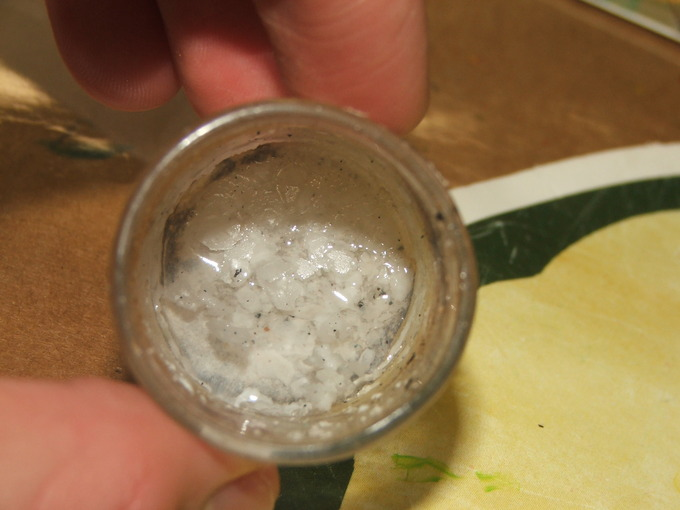## The Common Ion Effect

#### Learning Objective

• Explain the common ion effect.

#### Key Points

• The role that the common ion effect plays in solutions is mostly visible in the decrease of solubility of solids. Through the addition of common ions, the solubility of a compound generally decreases due to a shift in equilibrium.
• The common ion effect also plays a role in the regulation of buffers. Buffering solutions contain either an acid or base, accompanied by its conjugate counterpart. Addition of more like conjugate ions will ultimately shift the pH of the solution.
• The common ion effect must be taken into consideration when determining solution equilibrium upon addition of ions that are already present in the solution.

#### Terms

• Le Chatelier’s principleThe principle used to predict the effect of a change in conditions on a chemical equilibrium.
• bufferA solution used to stabilize the pH (acidity) of a liquid.
• conjugate baseThe species that is created after the donation of a proton.
• conjugate acidThe species created when a base accepts a proton.

## Solubility

Solubility refers to the amount of material that is able to be dissolved in a particular solvent. For example, table salt (NaCl) placed in water eventually dissolves. However, if more table salt is continuously added, the solution will reach a point at which no more can be dissolved; in other words, the solution is saturated, and the table salt has effectively reached its solubility limit.

Chemical equilibrium is the chemical state where there are no net physical or chemical changes between the reactant and the products of a reaction. This is because the rate of the forward (reactant to product) and reverse (product to reactant) reactions are equal.

Solubility equilibrium refers to the state of chemical equilibrium between a chemical compound in the solid state and a solution composed of that dissolved compound. This equilibrium is established when the rates of migration between the solid and aqueous phases of the molecules (or ions) are equal.

## Common Ion Effect

Whenever a solution of an ionic substance comes into contact with another ionic compound with a common ion, the solubility of the ionic substance decreases significantly. For example, this would be like trying to dissolve solid table salt (NaCl) in a solution where the chloride ion (Cl) is already present. The amount of NaCl that could dissolve to reach the saturation point would be lowered. This phenomenon is the common ion effect and plays important roles in pharmaceutical and environmental areas. The common ion effect can be explained by Le Chatelier’s principle of chemical equilibrium:

$AB_{(s) }\leftrightarrow { A^+ }_{ (aq) } + { B^-}_{ (aq) }$

For a simple dissolution process, the addition of more of one of the ions (A+) from another compound will shift the composition to the left, reducing the concentration of the other ion (B), effectively reducing the solubility of the solid (AB). For example, when calcium fluoride dissolves into calcium and fluoride ions, the solubility product expression is:

$CaF_{2(s) }\leftrightarrow { Ca^{+2} }_{ (aq) }+{ 2F^{-} }_{ (aq) }$

This expression must always hold, even if some ionic species come from other sources. Therefore, if more $Ca^{+2}$ ions are placed in solution, the equilibrium will shift to the left, favoring the solid form and decreasing the solubility of the solid.Lithium hydroxide with carbonate growthsLithium hydroxide forms less-soluble lithium carbonate, which precipitates because of the common ion effect.

## Buffer Solutions

A buffer solution is composed of a weak acid and its conjugate base, or a weak base and its conjugate acid. Addition of excess ions will alter the pH of the buffer solution. Therefore, the common ion effect takes a role in pH regulation. According to Le Chatelier’s principle, addition of more ions alters the equilibrium and shifts the reaction to favor the solid or deionized form. In the case of an an acidic buffer, the hydrogen ion concentration decreases, and the resulting solution is less acidic than a solution containing the pure weak acid.

For example in the reaction:

$HCN_{(aq)}\leftrightarrow {H^+}_{(aq)} + {CN^-}_{(aq)}$

The addition of cyanide ions (CN) will suppress the ionization of hydrogen cyanide (HCN) and shift its equilibrium to the left. The percent dissociation of the hydrogen cyanide will decrease, therefore decreasing the H+ ions and increasing the pH of the solution.